## 2006年5月24日 星期三

### 清明時節雨紛紛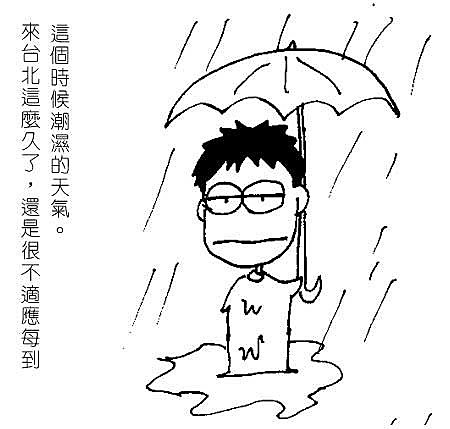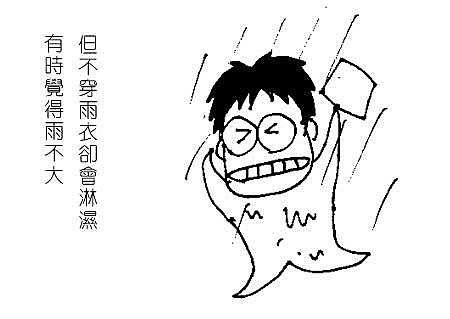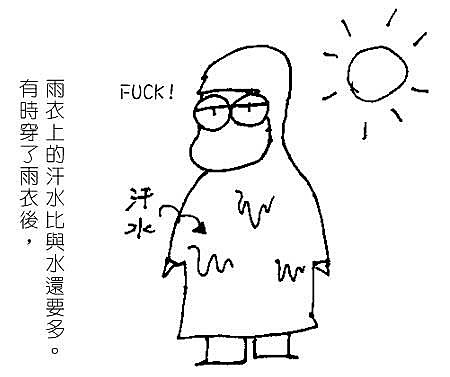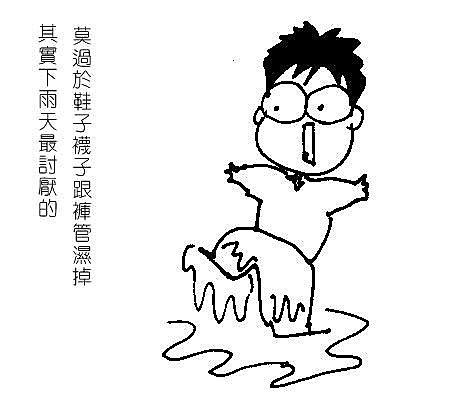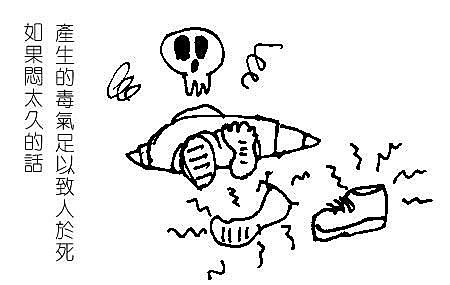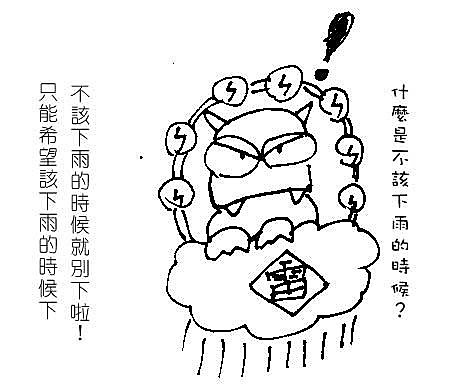#### 52 則留言:

1.椰~
我第一XD
我要太陽呀~(吶喊)

2.我也蠻討厭下雨的..
我比較喜歡晴天~^^~

3.:)) 說得是耶! =D>

4.應該說下在該下的地區就好 !
水庫集水區缺水...市區積水可以划船... :))

5.♀﹏流浪小藍﹏♂2006年5月25日 上午10:02

今天天氣很好~~應該不會下雨了吧!! ㄏㄏ

6.氣象局報導的一向不是台北氣象嗎？
說下雨就下雨～只是多大不能預測！
台中的天氣卻是說下雨沒下，還豔陽高照～
而豔陽高照，就一定來個午後雷陣雨！ :-O

7.在很久以前，曾有ㄧ陣子的天氣也是陰晴不定，
每次我只要有帶傘去學校就ㄧ定不會下雨，但沒帶時它就會給我下........
所以那陣子朋友都會問我：你明天有要帶傘嗎？（你帶我就不帶=____=）
唉......說起來，也已經是高中時的事了呢（喝茶） ←果然是很久以前啊= ="

8.呵呵~~好有趣！ =D>

9.哈…習慣就好啦~~台北就是這樣呀~~~要下不下，要大不大~~~記得隨身帶雨具就好了~~~若是騎車就記得在車箱裡放一件雨衣，寧可穿著也不要被淋溼吧~~

10.真想問天上的神決定好了沒有?
老是搞不定  真討厭

11.其實老天爺也很為難的~

亮仔~你忍耐一下吧!
謝謝你的來訪!
第一次來訪~ 你真有趣........   :">

12.小蘋果-哈哈琪2006年5月25日 下午1:45

=D> 你畫的真好!

13.跟我希望的一樣
怕一直沒下雨就會停水.很麻煩很不舒服的
所以就讓雨在我在家時下吧
哈哈...

14.語嚇得這樣好
圖下雨都詩意

15.哈 :)) 好有趣的雷公樣~!

16.氣象局如果說會下雨就不用帶雨具....
氣象局如果說會放晴那就要帶雨具....
提供給你參考一下....不過我的預測天氣的準度跟氣象局不相上下..... [-(

17.小丫頭‧°εїз°．﹒‧°2006年5月25日 下午5:09

嗯~台北比較常下雨，台中倒是還好囉~ :))

18.好好笑ㄛ~~真是寫實ㄉ圖~哈哈~可愛

19.:)) 說的好 :))

20.哈哈~真是有趣，如果雨都能下在水庫就好了。 ;)

21.有雨當思無雨之苦~~~大家共勉之!!!

22.第一次造訪，班大貓圖文並茂，非常吸引人，不過，回應時有一點小猶豫，~~~只約老人~~~~回應不就洩了底？唉！我的青春小鳥一去不回來！反正，老又不是我的錯，我來啦！
大貓的建議堪稱破天荒創舉，今年年底土地公回天庭述職時會提報辦理，以後下雨可望按尖峰時間和離峰時間分配雨量，必要時也可以考慮彈性調整，一切以便民為要！

23.我也常遇到這種是ㄝ！真是氣人，不過沒有毒氣可以逼人啦！而是我的襪子都發霉了！〈誇飾法....誇飾法你知道嗎？〉

24.:)) :)) :)) :)) :)) :)) :)) :)) :)) :)) :)) :)) :)) :)) :)) :)) :)) :)) :)) :)) :)) :)) :)) :)) :)) :)) :)) :)) :)) :)) :)) :)) :)) :)) :)) :)) :)) :)) :)) :)) :)) :)) :)) :)) :)) :)) :)) :)) :)) :)) :)) :)) :)) :)) :)) :)) :)) :)) :)) :)) :)) :)) :)) :)) :)) :)) :)) :)) :)) :)) :)) :)) :)) :)) :)) :)) :)) :)) :)) :)) :)) :)) :)) :)) :)) :)) :)) :)) :)) :)) :)) :)) :)) :)) :)) :)) :)) :)) :)) :)) :)) :)) :)) :)) :)) :)) :)) :)) :)) :)) :)) :)) :)) :)) :)) :)) :)) :)) :)) :)) :)) :)) :)) :)) :)) :)) :)) :)) :)) :)) :)) :)) :)) :)) :)) :)) :)) :)) :)) :)) :)) :)) :)) :)) :)) :)) :)) :)) :)) :)) :))

:))
:))

25.現在有一種雨衣
聽說很貴
它可以從頭到腳包好好...
公事包帶之摺疊傘吧
不然就是小雨戴帽子囉
台北的雨應該都是酸的...
天空可黑的很勒~

26.你有沒有試過穿雨鞋.....=___=|||
上次我看到一個外國人穿~~挺有趣的~

27.>:) ...    這篇的線條有比較簡單呦~~
是搭捷運的時候畫的嗎 ?!  ( 草率 ) :>

but~   內容可是符合了所有大台北人的心聲.. =D>

28.:)) 身為女性在冬季可拿靴子當雨鞋應急用!!但是夏季只能認栽!讓腳丫丫任雨肆虐囉!! >:P

29.:)) 呵呵~~~

30.:D 我也希望我載小孩上下學時別下雨.其他時間隨便啦!

31.你的漫畫好好笑喔^^"
我是希望凌晨的時候下雨，
因為聽著滴答滴答的雨聲和轟轟的打雷聲真的是一種享受!!! :P

32.O:-) 現實與幻想交替著~ >:)
你真ㄉ粉利害喔~ =D>
加油喔 !! :D

33.我覺得你是雨神喔
不如你出門的時候說一聲
我才帶雨傘 :))

34.嗯,我希望祂在我睡覺時下雨
涼涼爽爽超好睡的,
尤其是現在,昨天還下雨,今天下班怎出個超級大太陽,
厚!!粉熱餒~~~一定睡不好

35.渴望新生活 MY LIFE2006年5月26日 中午12:31

哈哈  對呀 在上下班擠公車的時候還下雨
那種感覺真的很討厭呢
到處都是濕答答的感覺 B-)

36.嗯．．．．．．．．．
每到這種雨天，而我又必須穿上雨衣騎機車時
我就會瞬間化為「盡禪人」

37.呵.....北部本來就是不穩定的天氣居多麻......
尤其端午節還沒過
動不動就來個午後雷陣雨....
等到炎夏真正來臨時
你就會懷念下雨囉~

38.離開台北這麼久了..
還真想念午後的雷陣雨
站在落地窗前面
看著台北再被洗滌
真希望你能畫一副思念的圖
看到你在台北的不習慣
倒讓我這台北人想念起故鄉了呢

39.落葉它為什麼落下,它自己也不懂2006年5月27日 晚上10:03

:)) :)) :))

40.當我決定帶雨具的時候祂就不下雨
反而決定不帶雨具的時候祂就偏要下雨… T_T

41.過期海苔仙貝2006年5月28日 下午2:35

我也超討厭下雨的說..

42.8-| 是阿
現在的天氣
不是我愛說的
真的讓人感到不悅
熱就熱到快溶化
說下雨就下雨
搞什麼!!

43.愛抬槓ㄉ晧媽2006年6月6日 下午4:08

對阿~每次下雨我都在想..拜託!不要在上下班時間下啦!!

44.不想下時就吊個晴天娃娃吧!
哆啦A夢裡的大雄都這麼做.....^^

45.愛上雨天的女孩2006年6月8日 上午10:13

你真ㄉ很口愛ㄋㄟ~~~~ :)) :))

46.:)) :)) :)) 最近一定得穿才行~~~~ :)) :)) :))

47.:( 身上穿了三四十年,超透明,超透氣的雨衣 :-S 下雨就給它衝過去

48.哈哈~~看完倪ㄉ插圖心情都不下雨了~~ :))

49.我最喜歡下雨了,可是台中很少下雨。

50.世界的溫度決定於青年的一念2006年7月8日 下午2:15

:))

51.極簡生活，讓自己更輕鬆！2007年10月9日 下午2:21

我也討厭下雨，不習慣台北陰雨的天氣

1.事啊!!!!我來台北都十多年了，一直沒有習慣這鬼天氣!!!